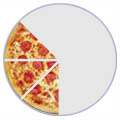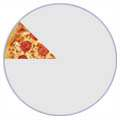# 带分数乘法

（"带分数" 也叫 "混合数"）

1. 转换成 假分数
2. 乘分数
3. 把结果转换回带分数

## 例子： 1 3/8 × 3 是什么？1 3/8 是一个披萨和另一个的八分之三。把披萨切开八份，每份八分之一，总共有几个八分之一？ 一整个切开来的8个，加上本来的三个八分之一 = 8+3 = 11 个八分之一。1 3/8 × 3 = 11/8 × 3/1 = 33/8 你有 33 个 八分之一。33个 八分之一 是 4个披萨 （4×8=32） 和剩下 1 个 八分之一。

1 3/8 × 3 = 11/8 × 3/1 = 33/8 = 4 1/8

## 例子： 1 1/2 × 2 1/5 是什么

1. 转换为假分数
2. 乘分数
3. 把结果转换回带分数### 一步一步来做：

1 1/2 × 2 1/5 = 3/2 × 11/5

3/2 × 11/5 = (3 × 11)/(2 × 5) = 33/10

33/10 = 3 3/10

1 1/2 × 2 1/5 = 3/2 × 11/5 = 33/10 = 3 3/10

## 再来一个例子： 3 1/4 × 3 1/3 ？

3 1/4 × 3 1/3 = 13/4 × 10/3

13/4 × 10/3 = 130/12

130/12 = 10 10/12 = 10 5/6

3 1/4 × 3 1/3 = 13/4 × 10/3 = 130/12 = 10 10/12 = 10 5/6

## 这个有负数： -1 5/9 × -2 1/7 是什么？

1 5/9 = 9/9 + 5/9 = 14/9
2 1/7 = 14/7 + 1/7 = 15/7

-14/9 × -15/7 = -14×-15 / 9×7 = 210/63

210/63 = 30/9 = 10/3

10/3 = (9+1)/3 = 9/3 + 1/3 = 3 1/3